omG8HI9LSKFW6lhCj8prTs0Z6lUhdOT9Jhi1Sf4m

# Sinusoidal or Sine Waveform : Basic Theory of AC Circuit

Sinusoidal wave is one of the most important waveform in Electrical Engineering. After many explanation about dc circuit, let us move on to the next level material. In electrical circuit, there will be two types of the electric sources : ac (alternating current) and dc (direct current). Make sure to read the dc circuit first in order to understand next explanation. Because up until now we limit the electric circuit basic theory only using dc circuit.

## What is Sinusoidal Wave

Before we start, why we need to learn ac circuits? Although it is harder to learn, it comes with many benefits. For history lesson, dc sources were the primary electric sources until late 1800s. In the beginning of the next century, the alternating current was introduced. Those two had a quite battle among the engineers. Because ac has more efficiency and better economic transmission, thus it ended up as a winner.

Sinusoidal source is useful for ac circuit as the same with the dc source important role for dc circuit.

After we studied about constant value of electricity over time, we will study about time-varying voltage and current. First thing, we will give our focus on sinusoidal electricity waveform or simply sinusoid.
sinusoid is a signal waveform which has the form of cosine or sine function.
For most of the time, alternating current (ac) refers to sinusoidal waveform. Sinusoid means the value has positive and negative values in spesific interval. An ac circuit is a circuit which is driven by ac current or voltage sources.

To understand the ac circuit very well, you need to understand what is electric circuit first.

For a starting, why do we need to learn ac circuit? What is the "better" side of ac besides it is more complex than dc? The ac sources has some "better" reasons to be learned.

First is its characteristic as sinusoidal waveform. We often meet it in motion of an pendulum, vibrate a string, or some ripples on the water surface. Second, like we mentioned before, sinusoidal signal is easy to generate and transmit. The electricity supplied to the world, homes, factories, industries, laboratories, etc has sinusoidal waveform. Third, we can implement Fourier Analysis for analysis of periodic signals which has the characteristic of sinusoidal waveform. The last, it is quite complicated to understand, but sinusoid is easy to analyze because its integral and derivative functions are also sinusoids.

After so many benefits from learning sinusoidal, do you know what mathematical theory we will use for ac circuit? The most basic of the dc circuit analysis theory such as :
will be applied again for ac circuit. You need to understand some parameters found in ac circuit such as :
We will use the dc circuit analysis techniques for ac circuit such as :
Along with dc circuit theorems for ac circuit :

## Sinusoidal Function

Please look at sinusoidal function of ac voltage :(1)
where
Vm = amplitude of the sinusoid
ω   = angular frequency in radians/s
ωt  = the argument of sinusoid.

The sinusoidal waveform in Figure.(1a) shows a function of its argument and Figure.(1b) as function of time.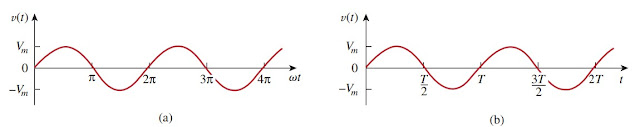Figure 1. Figure of Vm sin ωt : (a) in function ωt, (b) in function t.
From the figure, you will be able to grab the image of "repeat cycle" of sinusoid every T seconds, hence, T is called the period of the sinusoid. From two figures in Figure.(1), we conclude ωT = 2π,(2)
Notice v(t) has repetitive value every T seconds is shown by replacing t by t + T in Equation.(1). We obtain(3)
Thus,(4)
that, v has the same value at t + T same as when it is at t and v(t) can be called a periodic. In general,
periodic function is one that fulfills f(t) = f(t + nT), for all t and for all integers n.
T is different with t, where T is the time required for one cycle to complete or the number of seconds per cycle. T is also called by period. The opposite or reciprocal value of period (T) is frequency (f) of the sinusoid, means the number of cycles per second. Hence,(5)
From Equations.(2) and (5), we can conclude(6)
While ω is in radians per second (rad/s), f is in hertz (Hz).

Let us see a sinusoidal wave equation,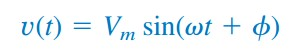(7)
where (ωt + ø) is the argument and ø is the phase. Both argument and phase can be in radians or degrees.
There are two sinusoids,(8)
shown in Figure.(2). The starting point of v2 in Figure.(2) occurs first in time. From Figure.(2) we can say that v2 leads v1 by ø or that v1 lags v2 by ø. If ø ≠ 0, we also say that v1 and v2 are out of phase. If ø = 0, then v1 and v2 are called to be in phase; they reach their minima and maxima at the same exact time. We are able to compare v1 and v2 in this matter because they operate with same frequency even the amplitude is different. Below we will see how to graph sinusoidal functions.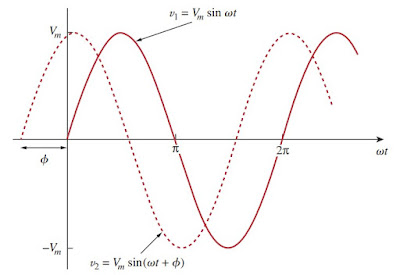Figure 2. Two sinusoids with different phases
Every sinusoid waveform can be expressed in either cosine or sine form. When comparing two sinusoids, it is wise to express in the same waveform as either cosine or sine with positive amplitudes. This can be achieved by using the following trigonometric function:(9)
Using these identities, it is easy to noticed that(10)
With these relationships, we can transform a sinusoid from cosine form to sine or vice versa.

A graphical drawing can be used to compare or relate as an alternative approach to using the trigonometric function in Equations.(9) and (10).Figure 3. Graphical (a) cos(ωt - 90o) = sinωt, (b) sin(ωt + 180o) = -sinωt
Notice the set of axes in Figure.(3a).
The horizontal axis refers to the magnitude of cosine and the vertical axis (points down) refers to the magnitude of sine. Angle is measured by positive value in counterclockwise direction from the horizontal axis.This calculation method can be used to compare two sinusoids.

For example, we consider in Figure.(3a) from subtracting 90o from cosωt results sinωt, or cos(ωt - 90o) = sinωt. Similarly, adding 180o to sinωt results -sinωt, or sin(ωt + 180o) = -sinωt, as can be seen in Figure.(3b).

This graphical method can add two sinusoids with the same frequency when one has sine waveform and the other has cosine waveform. In order to add A cosωt and B sinωt like shown in Figure.(4a), A is the magnitude of cosωt and B is the magnitude of sinωt.Figure 4. (a) Adding A cosωt and B sinωt, (b) Adding 3 cosωt and -4 sinωt.
Hence,(11)
where(12)
For simple example, we add 3 cosωt and -4 sinωt like shown in Figure.(4b) and results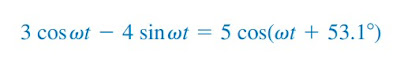(13)

## Example of Sinusoidal Function

For better understanding, let us review examples below :
1. Find the amplitude, phase, period, and frequency from
v(t) = 12 cos(50t + 10o)
Solution :
Amplitude : Vm = 12 V.
Phase : ø = 10o.
Angular frequency : ω = 50 rad/s/
Period : T = 2π/ω = 2π/50 = 0.1257 s.
Frequency : f = 1/T = 7.958 Hz.

2. Determine the phase angle between v1 = -10 cos(ωt + 50o) and v2 = 12 sin(ωt - 10o). State which one is leading.
Solution :
There are three ways to solve the problem. We can use trigonometric identities for the first two and use the graphical approach for the third.
Method 1v1 and v2 are in different form, so we need to make them have the same form, say cosine form.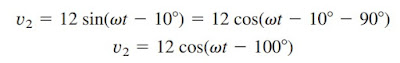(1.2)
It can be concluded from (1.1) and (1.2) that the phase difference between these two is 30o. We can rewrite v2 as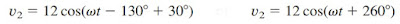(1.3)
Comparing (1.1) and (1.3) shows v2 leads v1 by 30o.

Method 2 : Let us express v1 in sine form
But v2 = 12 sin(ωt - 10o). Comparing these two shows v1 lags v2 by ωt, same with v2 leads v1 by 30o.

Method 3 : We may state v1 as simply -10 cosωt with +50o phase shift. We can draw v1 as in Fig.5.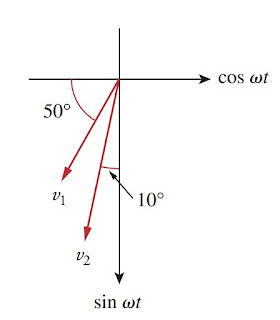Figure 5
Similarly, v2 is 12 sinωt with -10o phase shift as drawn in Figure.(5). We can conclude v2 leads v1 by 90o - 50o - 10o = 30o.

Untuk Bahasa Indonesia baca Rumus Gelombang Sinusoidal dan Contoh Soal.
Related Posts
SHARE